• matlab开发-图像局部缩放图像局部放大图像放大。这有助于将图像局部斑块放大为较大的斑块，以比较细节。
• ## Matlab图像局部放大

热门讨论 2011-02-22 23:16:47
实现了对Matlab绘制的图像局部放大，即呈现子图，以显示局部细节。
• 本算法简单的实现了图像局部放大的效果，算法需要确定放大区域的中心坐标点，以及放大圆形区域的半径和放大强度，来进行放大区域内的等比例放大，与美图秀秀的大眼功能类似。以人脸图像放大眼睛为例。在使用机器学习...
此代码可从https://github.com/TimeIvyace/Partial-Enlargement-of-Image.git中下载

本算法简单的实现了图像局部放大的效果，算法需要确定放大区域的中心坐标点，以及放大圆形区域的半径和放大强度，来进行放大区域内的等比例放大，与美图秀秀的大眼功能类似。以人脸图像放大眼睛为例。在使用机器学习等算法得到人脸68个特征点的基础上，我们可以大致确定眼珠中点的位置。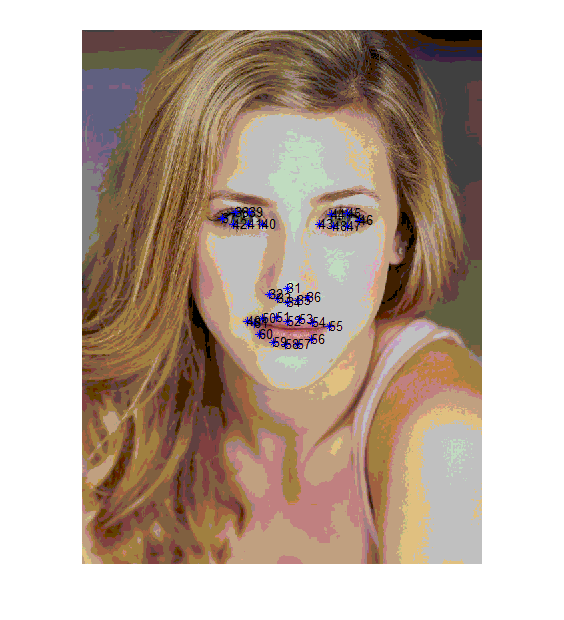由此可以大致得到左眼中心点位置为坐标37和40的中点，右眼中心点位置为坐标43和46的中点。接下来，通过设置放大区域半径以及放大强度，则可以实现眼睛放大。

局部放大算法代码：

function J=bigger(I,pointx,pointy,r)
%I为原图像，pointx和pointy为放大中心点坐标，r为放大半径
im=I;
%分别得到放大区域的上下左右坐标
left=round(pointy-r);
right=round(pointy+r);
top=round(pointx-r);
bottom=round(pointx+r);
%放大区域面积
space = r * r;
strength=30;    %放大强度
%原图像为彩色图像，要分成RGB三个分量进行处理
fr=im(:,:,1);
fg=im(:,:,2);
fb=im(:,:,3);
im2fr=fr;
im2fg=fg;
im2fb=fb;
%插值算法
for x=top:bottom
offsetx=x-pointx;
for y=left:right
offsety=y-pointy;
xy=offsetx*offsetx+offsety*offsety;
if xy<=space
%等比例放大
scale=1-xy/space;
scale=1-strength/100*scale;
%posy和posx为放大后坐标值
%采用最近邻插值算法
posy=round(offsety*scale+pointy);
posx=round(offsetx*scale+pointx);
im2fr(x,y)=fr(posx,posy);
im2fg(x,y)=fg(posx,posy);
im2fb(x,y)=fb(posx,posy);
end
end
end
%将RGB三个分量整合，得到彩色图像
J=cat(3,im2fr,im2fg,im2fb);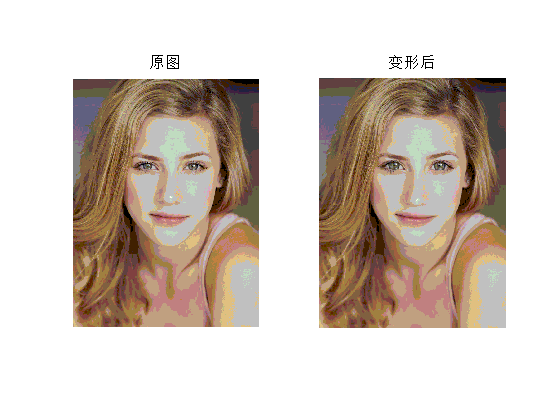算法没有对图像边界进行判断，若放大区域超出图片范围，则会出错。插值算法使用的是最简单的最近邻算法，所以放大区域边界并不是特别平滑，接下来可以使用更高阶的插值算法进行改进。通过修改放大半径r以及放大强度strength，可以得到一个较为满意的效果。
展开全文• 投稿科技论文 SCI 作图必备 技能之 MATLAB局部放大图像的 画法等命令连魏魏 科技论文 SCI 作图必备技能之 MATLAB局部放大图像的画法 等命令连魏魏 科技论文写作时 常常碰到图中某个局部太小看不到的情 况这时你可以...
• matlab_图像局部放大 。。。 H=axes(); x3=time(x1:x2);y3=series(x1:x2); plot(x3,y3); set(H,'position',[0.3 0.65 0.2 0.15]);
• MATLAB 仿真图像局部放大 相信是很多学习MATLAB学习者必备的 呵呵 从别人那拷过来的资源
• 图像剪裁和bicubic插值放大，直接放代码 clc;clear; close all; im = imread('C:\Users\Administrator\Desktop\2.png'); X = 527; %矩形框左上角的横坐标 Y = 257; %矩形框左上角的纵坐标 dX = 147; %矩形框的宽 ...
图像剪裁和bicubic插值放大，直接放代码
%
代码用途：
图像剪裁和bicubic插值放大，局部放大显示
%
clc;clear;
close all;
im = imread('C:\Users\Administrator\Desktop\2.png');

X    = 527; %矩形框左上角的横坐标
Y    = 257; %矩形框左上角的纵坐标
dX   = 147; %矩形框的宽
dY   = 137;  %矩形框的高
bbox = [X, Y, dX, dY];
im_1 = insertShape(im, 'Rectangle', bbox, 'LineWidth', 2,  'Color', 'green');%在im中画出bbox，矩形框，边框线粗2，颜色为绿色
imshow(im_1);%显示im_1
imwrite(im_1,'C:\Users\Administrator\Desktop\1a.png');%存储im_1

%% 裁剪和局部并插值放大
scale     = 4;%放大尺度
im_crop   = imcrop(im, bbox); %从图像im中剪裁出bbox
imshow(im_crop);%显示im_crop
imwrite(im_crop,'C:\Users\Administrator\Desktop\1b.png');%存储im_crop

im_crop_b = imresize(im_crop, scale, 'bicubic'); %对剪裁的图像使用bicubic放大
imshow(im_crop_b);%显示im_crop_b
imwrite(im_crop_b,'C:\Users\Administrator\Desktop\1c.png');%存储im_crop_b

%% 局部显示
[row_1, col_1, ~] = size(im);%获取图像的尺寸
[row_2, col_2, ~] = size(im_crop_b);%获取剪裁放大后的图像的尺寸
im_2= im_1;%将在im中画出bbox框的图像复制给im_2
im_2(row_1-row_2+1:col_1, 1:col_2, :) = im_crop_b;%将im_crop_b图像内容复制给im_2图像左下角的位置
bbox1  = [1,row_1-row_2+1,col_2,row_2];
im_2  = insertShape(im_2, 'Rectangle', bbox1, 'LineWidth', 2, ...
'Color', 'blue');%在im_2中画出bbox1，矩形框，边框线粗2，颜色为蓝色
figure;imshow(im_2);
imwrite(im_2,'C:\Users\Administrator\Desktop\1d.png');%存储im_2



展开全文• 20121219如何在Matlab画图时，放大一幅图中的局部细节写论文的时候，有时需要放大曲线图中的一部分以使图像细节更明显，便于比较不同的曲线结果。同时这也可以使得自己的曲线图看起来更美观。这里介绍一种比较有效的...
20121219如何在Matlab画图时，放大一幅图中的局部细节写论文的时候，有时需要放大曲线图中的一部分以使图像细节更明显，便于比较不同的曲线结果。同时这也可以使得自己的曲线图看起来更美观。这里介绍一种比较有效的方法。主要运用的函数为：axes('position',[0.2,0.55,0.1,0.1])其中[0.2,0.55,0.1,0.1]是所画的小图的位置，表示以整个画图板([0,0,11])为参考，左下角的坐标为(0.2，0.55)，长和宽分别为0.1 0.1。以下是举例代码：(其中的数据为自己论文中用的数据)：%载入数据load('cf.mat')load('Regular.mat')load('ref.mat')%画图hold ontn=1:1500; %横坐标plot(tn, A_ref,'k');plot(tn, A_cf,'r-.');plot(tn,A_Regular,'b:');legend('Enlarged domain','C-PML','Regular PML')得到的我们的初始图为：%如果想放大在(250——600)中的数据，可添加如下语句实现。%小图的坐标控制范围M1=250;
展开全文• 最近使用MATLAB图像处理方面的GUI开发，现在需要将axes1里的原图像局部进行放大并在axes2里进行显示。 为了美观，现在设置两个坐标系相同大小。可否能像ENVI软件那样，在原图上有一个固定大小的滑动窗口，...开发语言
• 效果 鼠标选择bounding box 左上角位置 ...以图片1.jpg为例，原图存放在root文件夹，用3种方法的处理结果存放在method1，method2，method3子文件夹，matlab代码drawcompare.m放在root文件夹。 ——root：1.jp...
效果

鼠标选择bounding box 左上角位置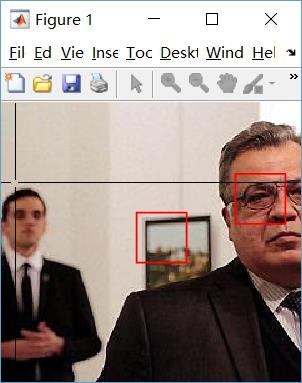生成的图。该脚本以bounding box大小为基准，调整大图的大小。贴到论文里比较好看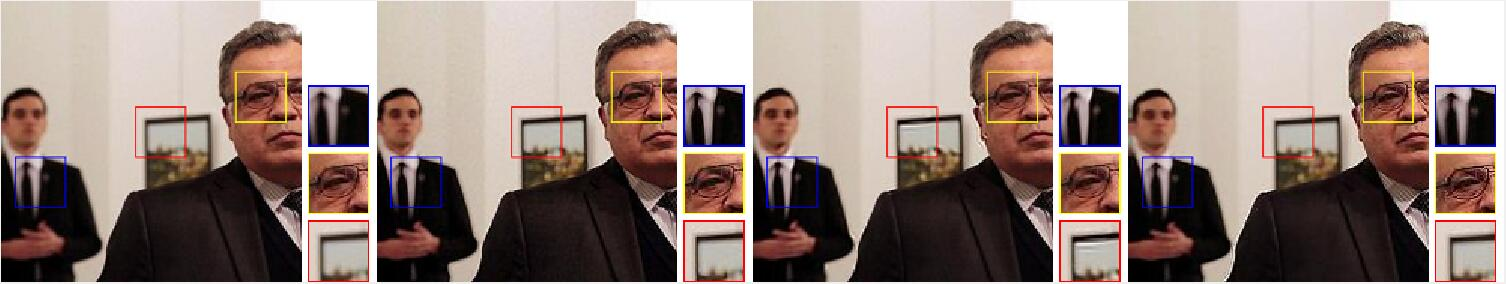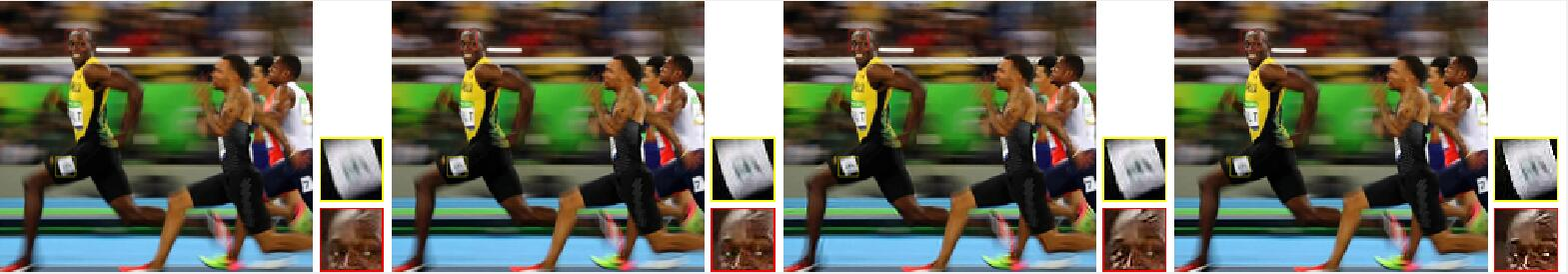文件存放形式

以图片1.jpg为例，原图存放在root文件夹，用3种方法的处理结果存放在method1，method2，method3子文件夹，matlab代码drawcompare.m放在root文件夹。

——root：1.jpg drawcompare.m
|————method1: 1.jpg
|————method2: 1.jpg
|————method3: 1.jpg


代码

drawcompare.m

用法：
1. 按照文件存放形式放好待比较图像
2. 运行函数代码（若报错“方框数量太多”，请减少方框数量，重新运行函数）

 函数参数分别为：
imname：图像文件名
fixw：（正方形）方框边长（默认为40）
margine：留白大小（默认为10）
nbox：方框数量（默认为2）


3. 点击图像选择方框左上角，按下回车确认（若报错“超出了图像范围”，请重新运行函数，小心的选择位置）
4. 生成的图像会保存在该代码所在目录

function []=drawcompare(imname,fixw,margine,nbox)
%input:
% imname: string image filename eg: 'street.jpg'
% fixw: bounding box size for zoom in
% margine: margine between picture elements
% nbox: number of bounding boxs
%usage:
% 1.put the origine image in the root dir
% 2.put the images (to be compared, having same file name as the origine
% image) in different sub dir,
% 3.click the image and press enter to confirm your bounding boxs （2 box in
% this program）
% 4.new image will be save to root dir

%------------------sub dir, modify by user---------------------------------
me_dir = './ME';
pan_dir = './PAN';
xu_dir = './XU';
sun_dir = './SUN';
ori_dir = '.';
method_dir_list={ori_dir,me_dir,pan_dir,sun_dir,xu_dir};

%-----------------default params-------------------------------------------
if (nargin<2 || fixw<=0)
fixw=40; margine=10;
end
if (nargin<3 || margine<=0)
margine=10;
end
if (nargin<4 || nbox>6)
nbox=2;
end

%------------------计算各种大小--------------------------------------------
% 这里设resize后发img宽为patch的scale倍，scale=5
scale=5；
imme = imread(fullfile(me_dir,imname));
[h,w,c]=size(imme);
imme_re = imresize(imme,[NaN,fixw*scale]);
[hr,wr,~]=size(imme_re);
assert(hr>fixw*nbox+(nbox-1)*margine/2,'error: too many nbox!')
wrr = wr+margine+fixw;% width + pad + patch
imall_final = 255*uint8(ones(hr,length(method_dir_list)*(wr+margine+fixw),3));
imone_final = 255*uint8(ones(hr,wr+margine+fixw,3));

%-------------------获取框的位置 set boundingbox---------------------------
figure(1)
axis image;
set(gcf,'WindowStyle','normal')
set(gcf,'unit','pixels','Position',[300,300,w,h]);
imshow(imme,'border','tight','initialmagnification','fit');  %图像填充figure
point_cell={};
for i=1:nbox
[x,y]=ginput;
x=int32(ceil(x(end)));y=int32(ceil(y(end)));
rectangle('Position',[x y fixw fixw],'LineWidth',1,'EdgeColor','r')
point_cell{i}=[x,y];
end
%------------------在原图画框 draw boundingbox-----------------------------
colors=lines(nbox);
% red yellow blue pink cyan orange

for i=1:length(method_dir_list)

im = imread(fullfile(method_dir_list{i},imname));
im_rect = im;
for j=1:length(point_cell)
x = point_cell{j}(1);
y = point_cell{j}(2);
im_rect=drawRect(im_rect,[x,y],[fixw,fixw],1,color(j,:);
% 裁剪框的部分
im_crop=imcrop(im_rect,[x y fixw fixw]);
% 调整原图大小 贴到最终图
imone_final(hr-j*fixw-(j-1)*margine/2:1:hr-(j-1)*fixw-(j-1)*margine/2,...
wr+margine/2:1:wr+margine/2+fixw,:) = im_crop;
end
imone_final(1:1:hr,1:1:wr,:) = imresize(im_rect,[hr,wr]);
imall_final(:,(i-1)*wrr+1:1:i*wrr,:)=imone_final;
end

figure(2),imshow(imall_final)
imwrite(imall_final,['all' imname]);

end
function [ dest ] = drawRect( src, pt, wSize,  lineSize, color )
% 转载自 http://blog.csdn.net/humanking7/article/details/46819527
% 简介：
% %将图像画上有颜色的框图，如果输入是灰度图，先转换为彩色图像，再画框图
% 图像矩阵
% 行向量方向  是  y
% 列向量方向  是  x
%----------------------------------------------------------------------
%输入：
% src：        原始图像，可以为灰度图，可为彩色图
% pt：         左上角坐标   [x1, y1]
% wSize：   框的大小      [wx, wy]
% lineSize： 线的宽度
% color：     线的颜色      [r,  g,  b]
%----------------------------------------------------------------------
%输出：
% dest：           画好了的图像
%----------------------------------------------------------------------

%flag=1: 有缺口的框
%flag=2: 无缺口的框
flag = 1;

%判断输入参数个数
if nargin < 5
color = [255 255 0];
end

if nargin < 4
lineSize = 1;
end

if nargin < 3
disp('输入参数不够 !!!');
return;
end

%判断框的边界问题
[yA, xA, z] = size(src);
x1 = pt(1);
y1 = pt(2);
wx = wSize(1);
wy = wSize(2);
if  x1>xA || ...
y1>yA||...
(x1+wx)>xA||...
(y1+wy)>yA

disp('画的框将超过图像 !!!');
return;
end

%如果是单通道的灰度图，转成3通道的图像
if 1==z
dest(:, : ,1) = src;
dest(:, : ,2) = src;
dest(:, : ,3) = src;
else
dest = src;
end

%开始画框图
for c = 1 : 3                 %3个通道，r，g，b分别画
for dl = 1 : lineSize   %线的宽度，线条是向外面扩展的
d = dl - 1;
if  1==flag %有缺口的框
dest(  y1-d ,            x1:(x1+wx) ,  c  ) =  color(c); %上方线条
dest(  y1+wy+d ,     x1:(x1+wx) , c  ) =  color(c); %下方线条
dest(  y1:(y1+wy) ,   x1-d ,           c  ) =  color(c); %左方线条
dest(  y1:(y1+wy) ,   x1+wx+d ,    c  ) =  color(c); %左方线条
elseif 2==flag %无缺口的框
dest(  y1-d ,            (x1-d):(x1+wx+d) ,  c  ) =  color(c); %上方线条
dest(  y1+wy+d ,    (x1-d):(x1+wx+d) ,  c  ) =  color(c); %下方线条
dest(  (y1-d):(y1+wy+d) ,   x1-d ,           c  ) =  color(c); %左方线条
dest(  (y1-d):(y1+wy+d) ,   x1+wx+d ,    c  ) =  color(c); %左方线条
end
end
end %主循环尾

end %函数尾



Ref

画框函数：[图像]用Matlab在图像上画矩形框
展开全文• %构造一个新的坐标轴/画图区域（局部放大图像） %[x1,x2,y1,y2]是新坐标轴原点位于原图中的位置点（按比例） plot(x1,y_negative,'r',x2,y_positive,'g'); axis([0,30,0,0.6]); %作图并限定坐标轴的范围，实现图像...
• 一、课题介绍matlab具有完备的图形处理功能、友好的用户界面以及功能强大的图形处理工具箱，能够实现对数字图像的编辑和处理工作，实现功能包括数字图像的读取、存储、显示、去色、图像翻转、局部放大、透明度调整、...
• Matlab图形需要对某个局部区域进行放大，通过鼠标点击方式选择区域，并将放大的区域图形置于图像的右下角，便于图像对比观察。
• 图像中用于放大图像细节做对比试验的matlab代码，代码实现了可以用鼠标在图像中选取任意区域大小进行指定数值倍数的放大
• Matlab中对实验结果进行局部放大图像去噪研究中，通常使用SNR, PSNR, MSE, and SSIM等标准去定量衡量去噪的效果，然而，这是需要原图作为benchmark的，在实际获取的图像中，不可能得到原图，这时对去噪效果的...数据可视化 计算机视觉
• 转自：... 网上看到的matlab局部放大代码，认真看了看，学了不少东西。 %%%%%%%%%%%%%%%%%%%%%%%%%%%%%%%%%%%%%%%%%%%%%%%%%%%%%%%%%%%%%%%%%%%%%%%%%%%%%%%%%%%%%%%%%%%%%%%%%%%%
• 课题介绍matlab具有完备的图形处理功能、友好的用户界面以及功能强大的图形处理工具箱，能够实现对数字图像的编辑和处理工作，实现功能包括数字图像的读取、存储、显示、去色、图像翻转、局部放大、透明度调整、去噪...
• clc,clear img=imread('C:\1.jpg'); pos=[3 3];%起始点不能放在[1 1] siz=[99,79];%画框坐标和尺寸，[宽，...%调用函数(图像，左上角起点坐标，尺寸，线宽，颜色) i_zoom=imcrop(I1,[pos,siz]);%裁剪出框中局部图 ...经验分享
• matlab数字图像处理系统，实现功能包括数字图像的读取、存储、显示、去色、图像翻转、局部放大、透明度调整、去噪、平滑、锐化、压缩、边缘检测等操作。
• 课题介绍matlab具有完备的图形处理功能、友好的用户界面以及功能强大的图形处理工具箱，能够实现对数字图像的编辑和处理工作，实现功能包括数字图像的读取、存储、显示、去色、图像翻转、局部放大、透明度调整、去噪...计算机视觉 opencv python 机器学习 深度学习
• 科研必备之图像局部区域放大Matlab或QT实现）Matlab版本QT版本（含界面） 在撰写图像处理领域相关的论文中通常需要将图片的某个小块进行放大，以便论文审稿者看到图像清晰的细节信息，本文主要使用matlab和QT...Rect
• magnify.m 函数下载地址：magnify - File Exchange - MATLAB Central； magnify.m 函数在执行时，是一种交互式处理...% 加载图像到内存，如读取matlab自带的图像，并将其显示出来 I = imread('pout.tif'); imshow...
• %***彩色图像请用注释掉的部分***** Ic = imcrop(I,[x1 y1 x2 y2]); Is = imresize(Ic,s,'nearest'); %Ip = uint8([255*ones(size(I,1)+K1, size(I,2)+K2),3]); %for i=1:3 % Ip(:,:,i)=uint8(25
• matlab具有完备的图形处理功能、友好的用户界面以及功能强大的图形处理工具箱，能够实现对数字图像的编辑和处理工作，实现功能包括数字图像的读取、存储、显示、去色、图像翻转、局部放大、透明度调整、去噪、平滑、...
• 放大两张图像分析局部的情况。 图一 图二 图一为imshow显示局部内容，图二为imwrite保存后显示局部内容。可以看到图一保存后发生了模糊失真，原本边缘明显的噪点像素块边缘不再清晰。总体上来看就是模糊失真反而...# matlab放大局部图像matlab 订阅